Thursday , July 18 2019
Home » Igcse Mathematics Revision Notes » Set Language And Notation

# Set Language And Notation

### Set:

A set is a collection of objects, things or symbols which are clearly identified.

The individual objects in the set are called the elements or members of the set.

Elements may be specified in two ways:

• By listing the elements
• By description

E.g.

Listing                                                             Description

{2, 4, 6, 8, 10}                                                  The set of even numbers between 1 and 11

{a, e, i, o,u}                                                      The set of vowels in the alphabet

{} These braces stand for the word “the set of”

e.g.  {Even numbers between 1 and 11}

### Naming sets and number of members in a set:

Usually, we use Capital letters to denote a set and small letters to denote members of the set.

n ( ) indicates the total number of members in a set.

E.g.

A= {1, 3, 5, 7, 9, 11}      n (A) = 6

B= {2, 4, 6, 8}         n (B) = 4

### Membership of the set:

Є is an element of  (is a member of ) (belongs to)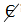is not an element of  (is not a member of) ( does not belongs to)

E.g.

A = {1, 3, 5, 7, 9, 11}

### Finite Sets:

Set in which all the elements can be listed.

A= {1,3,5,7,9}  n(A) =5

B= {days of the week beginning with T}  n(B) = 2

### Infinite Sets:

Sets in which it is possible to list all the members of a set.

C= {2, 4, 6, 8,10….}

E={x:x is a natural number}

### Universal Sets: (Ƹ)

The set which contains all the elements.

All proper subsets formed within the universal set draw their elements from the available elements of the universal sets.

### Complement Of a Set: (A`)

(Ƹ element – A element} = A`

If Ƹ = {2, 3, 5, 7, 11, 13}  and A = {2, 3, 7, 13}

A= {5, 11}

### Equal Sets:(C)

Two sets A and B are said to be equal if and only they have exactly the same elements.

Two equal sets are also subsets (denoted by C) of each other.

A={2,4,6,8}           B={8,6,2,4}

Then

A=B      B=A      A C B  B C A

### Subsets:

When each member of a set A is also a member of a set B, then A is a subset of B.

C is a subset of :When two sets have  exactly same elements or elements in the first set are also elements in the   second set.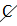is not a subset of: There is at least one element in the first set that does not belong to the second set.

### Proper Subset:

When each member of a set A is also a member of a set B, but set B has MORE elements than set A, then set A is a proper subset of B, denoted by “A C B”. Therefore, in this case set B is not a proper subset of A (BA)

C is a proper subset of: When each element in the first set also belongs to the second set, but the second set has more elements than the first set.

C is not a proper subset: When there is at least, one element in the first set that does not belong to the second set.

E.g. A = {1, 5, 9}     B = {1, 3, 5, 9}

Therefore:    A C B   , BA

### Empty Set: ({} or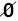)

A set which contains NO elements

An empty set in a subset of any set.

### Intersection Of Sets: (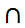)

Common elements in different sets.

A= {1,2,3,4,5,6}

B= {2,4,8,10}

Union Of Sets: U

The Union of set A and set B is the set of all elements which are in A, or in B, or in both A and B. It is denoted by ‘A U B’ and is read as “the union of A and B”.

A= {1,3,4}    B={6,7,8}

A U B = {1,3,4,6,7,8}

### Disjoint Sets:

If the two sets have No element in common then the two sets are called disjoint.

The intersection of two disjoint sets is null or empty.

e.g. A = {1,3,5,7}    and     B = {2,4,6,8,9}

De Morgans Law: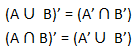### Venn Diagram:

In a venn diagram, we use a rectangle to denote a universal set Ƹ and a loop such as circle or an oval to represent any set in Ƹ .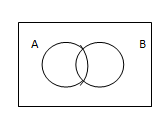Examples of Venn Diagrams: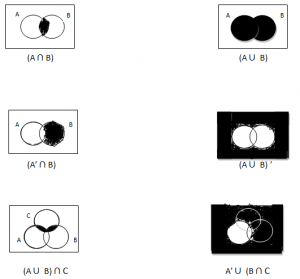## Properties Of A Circle

Circle Definitions: Geometrical Properties of Circle: If 2 chords in a circle area congruent, then …

### One comment

1.Hinah jawaid karim

Hi I am Hinah ! I’m thankful with useful pieces of material available at website. it really help to revise within limit of time as I am preparing for my final board exam, I would be grateful if you would get in touch with me as soon as possible. You can reach me on this email.